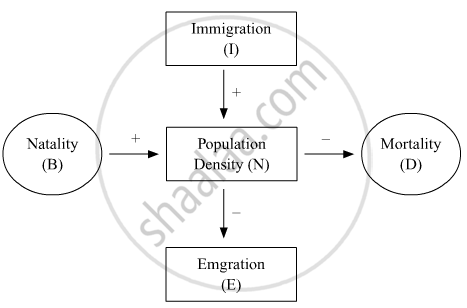# Study the flow chart given below and complete the equation that follows by identifying 1, 2, 3 and 4. Nt + 1 = Nt + {(1+ 2) − (3 + 4)} - Biology

Study the flow chart given below and complete the equation that follows by identifying 1, 2, 3 and 4.Nt + 1 = Nt + {(1+ 2) − (3 + 4)}

#### Solution

Nt + 1 = Nt + {(B+ I) − (D + E)}

Concept: Population - Population Attributes
Is there an error in this question or solution?